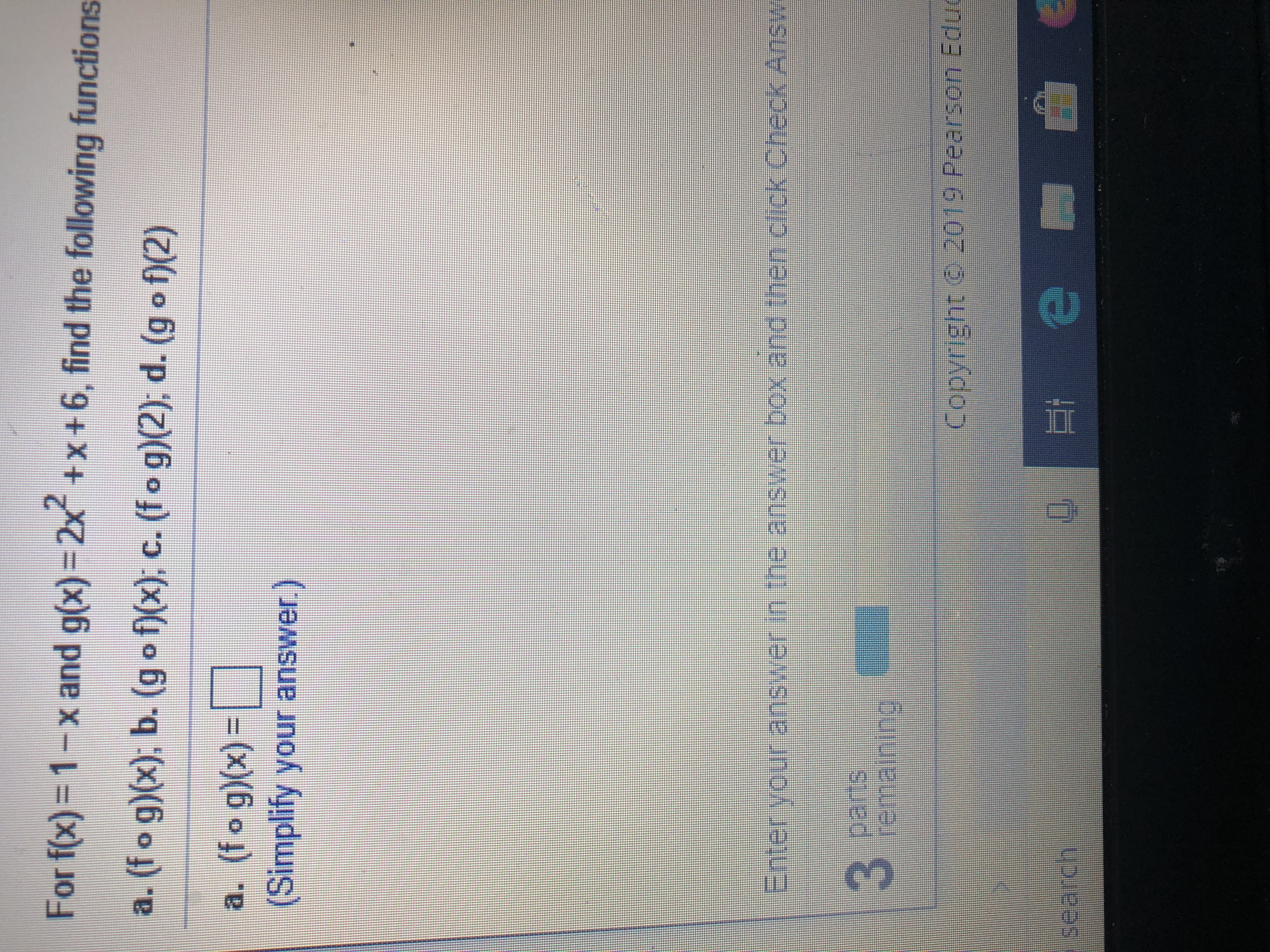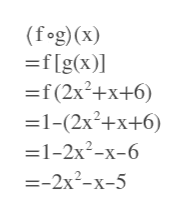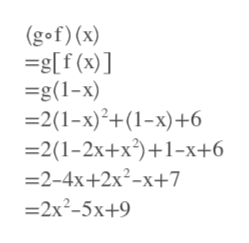For f(x) 1-x and g(x)= 2x +x+6, find the following functionsa. (f o g)(x): b. (gof)(x); c. (fo g)2), d. (g o f)(2)a. (fo g)x)(Simplify your answer.)ranser in the ansrer box and then cick check AnswerparisremainingCopyright @ 2019 Pearson Edu

Questionhelp_outlineImage TranscriptioncloseFor f(x) 1-x and g(x)= 2x +x+6, find the following functions a. (f o g)(x): b. (gof)(x); c. (fo g)2), d. (g o f)(2) a. (fo g)x) (Simplify your answer.) ranser in the ansrer box and then cick check Answ er paris remaining Copyright @ 2019 Pearson Edu fullscreen
Step 1help_outlineImage Transcriptionclose(fog) (x) =f[g(x)] =f (2x2+x+6) =1-(2x2+x+6) —1-2х*-х-6 --2x-х-5 fullscreen
Step 2help_outlineImage Transcriptionclose(gof) (x) -g[f (x)] =g(1-x) 2(1-x)2+(1-x)+6 2(1-2x+x21-x+6 -2-4x+2x2-x+7 -2x2-5x+9 fullscreen
Step 3

Want to see the full answer?

See Solution

Want to see this answer and more?

Our solutions are written by experts, many with advanced degrees, and available 24/7

See Solution
Tagged in

Other